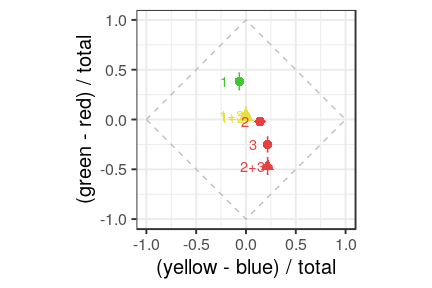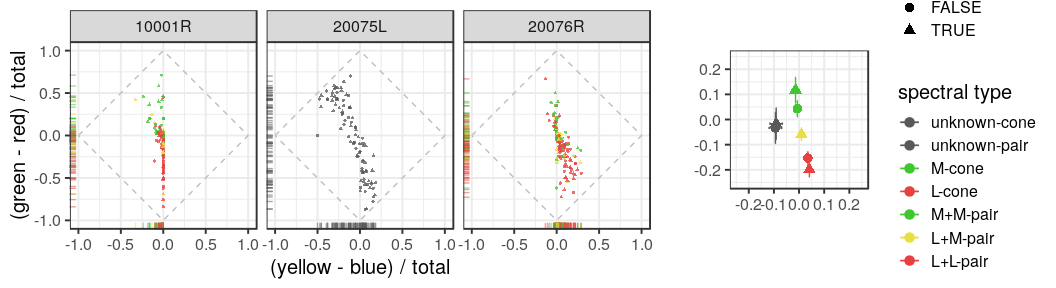Introduction

A central goal of vision science is to understand how signals from photoreceptors are transformed into sight and the limitations each stage of processing imposes on perception. Photoreceptors provide an organism with real-time information about the environment. However, the signals conveyed by individual neurons are noisy and ambiguous. One strategy for reducing uncertainty is to pool signals across multiple detectors. Under low light conditions, for example, the visual system combines signals from many hundreds of rod and cone photoreceptors in order to boost sensitivity . One drawback of signal pooling is a loss in spatial resolution: both acuity and contrast sensitivity are reduced under low-light levels . We studied the influence of spatial pooling on the color appearance of cone-targeted spots.

Results

First load in data and set some parameters that will be used during plotting later.

# setwd("~/R/Schmidt-Boehm-Tuten-Roorda_2019")
library(tidyverse)
library(ggthemes)
library(gridExtra)
library(corrplot)
library(car)
# set the colors that will be used in plots below.
red <- "#e84040"
green <-"#40c631"
blue <- "#4042e8"
yellow <- "#e8df40"
gray <- "#595959"
# make sure a figures directory exists
dir.create('figures', showWarnings=FALSE)

Example session

In a previous experiment, the one and two cone conditions were equated for detectability. We subsequently collected appearance measurements. All other stimulus conditions were identical to the detection task. Three cones were selected for study in each session (Fig~A). On each trial either a single cone or a pair was targeted. After each flash, the subject judged the color of the spot using a hue and saturation scaling paradigm . Each cone and pair was tested twelve times (72 trials per session). A total of 198 pairs were tested across three subjects. Hue and saturation scaling data were transformed into a color opponent representation. For each trial, the degree of perceived greenness versus redness and yellowness versus blueness was computed from percentage ratings as follows: \(gr = (green\% - red\%) / 100\%\) and \(yb = (yellow\% - blue\%) / 100\%\). In this representation, saturation is expressed as the distance from the origin (in city block metric). A pure white response falls at the origin and a maximally saturated report falls along the outer diamond.

The results of one session are plotted in Fig~B. In this example, Cone 1 was an M-cone and had a bias towards green. Cone 2 was an L-cone and elicited predominantly white reports. Cone 3, also an L-cone, was rated reddish-yellow (orange) with medium saturation. The motivation for this study was to understand the algorithm the visual system uses when combining information across cone pairs. In the example, when Cone 1 was targeted together with either Cone 2 or Cone 3, the average report was desaturated. In comparison, when Cone 2 and 3 were targeted they elicited a medium saturated orange report. Below, we analyze the results from all sessions and subjects.

example <- trial_data %>%
filter(., subject == '20076R', session== 4) %>%
group_by(., masterID1, masterID2, isPair) %>%
summarise(., yb_mean=signif(mean(yb), 3),
yb_sem=signif(sd(yb) / sqrt(length(yb)), 3),
gr_mean=signif(mean(gr), 3),
gr_sem=signif(sd(gr) / sqrt(length(gr)), 3), N=length(gr),
typeIDstr=typeIDstr)
boundary <- data.frame(x=c(-1, 0, 1, 0, -1), y=c(0, 1, 0, -1, 0))
(examplePlot <- ggplot(data=example,
aes(x=yb_mean,
y=gr_mean,
color=interaction(typeIDstr, isPair),
shape=isPair)) +
geom_point(size=3) +
geom_errorbar(aes(x=yb_mean, ymin=gr_mean - gr_sem, ymax=gr_mean + gr_sem)) +
geom_errorbarh(aes(y=gr_mean, xmin=yb_mean - yb_sem, xmax=yb_mean + yb_sem, height=0.01)) +
coord_equal(xlim=c(-1, 1), ylim=c(-1, 1)) +
xlab('(yellow - blue) / total') +
ylab('(green - red) / total') +
scale_color_manual(
values = c(red, green, red, yellow),
name="spectral type") +
geom_text(label=c('1', '1+2', '1+3', '2', '2+3', '3'),
nudge_x=-0.15) +
geom_path(data = boundary, aes(x=x, y=y), colour='gray', linetype=2, inherit.aes = F) +
theme_bw(base_size = 15) +
theme(legend.position="none")
)ggsave("figures/example_session.pdf")

Variability in sensations from cones with the same sensitivity

The motivating question behind these experiments was how does the visual system combine inputs from cones when making color appearance judgments? To begin to answer this question, we grouped each trial based on which cone or pair was probed. The results are reported in a table below. In both subjects with classified cones, individual and pairs of M-cones produced gr means that were greater than zero, while L-cone conditions led to negative values. This observation is consistent with a predictive relationship between cone type and color report, as found previously (Sabesan et al. 2006; Schmidt et al. 2018).

Each point in these plots represents the mean response measured from a single cone or a pair. This plot illustrates the variability in responses across cones/pairs and between subjects. There are a few features to note. Firstly, within a single subject, there was considerable variability between cones and pairs with the same spectral sensitivity. Similar variability in sensations from single cones has been reported previously (Hofer et al. 2005; Sabesan et al. 2016; Schmidt et al. 2018). This is the first report of variability in sensations elicited from pairs of cones. Secondly, there were individual differences in color responses: S20075 used blue more frequently than the two other subjects and S10001 did not report yellow on any trials. However, the general patterns are similar. Most of the variance was found along the green-red dimension; there were few points that fell in the blueish-red or greenish-yellow quadrants. In the two subjects with classified mosaics, we additionally found L-cone targeted trials tended produce a reddish bias, while M-cones were biased towards green. These patterns were similar to previous reports from single-cone (Sabesan et al. 2016; Schmidt et al. 2018) and large-field studies (DeValois et al. 1997).

To better appreciate the influence of cone type and number of cones targeted on color reports, data was pooled across subjects and grouped according to the type of cone or pair probed. The mean and standard error for each group is shown in the right most plot below. When an individual or pair of M-cones was targeted the average \(gr\) response was greater than zero indicating a bias towards green. In comparison, the average L-cone(s) elicited biases towards red and yellow. Together these cone type specific differences in color reports were consistent with a predictive relationship between cone type and color report, as previously reported (Sabesan et al. 2016; Schmidt et al. 2018). Two cones with the same photopigment tended to elicit slightly more saturated reports than single cone trials. On the other hand, one L- and one M-cone targeted together tended to produce desaturated reports.

(p <- grid.arrange(ind, avg, widths=2.5:1))
TableGrob (1 x 2) "arrange": 2 grobs
z     cells    name           grob
1 1 (1-1,1-1) arrange gtable[layout]
2 2 (1-1,2-2) arrange gtable[layout]Mosaic parameters do not predict responses

The plot above demonstrates that color reports varied even between cones with the same photopigment (Schmidt et al. 2018). Some L-cones, for instance, elicited highly saturated red sensations, while a majority produce white or desaturated pink reports. We next asked whether the variability in sensations between cones with the same sensitivity could be explained by low level features of the mosaic. Specifically, can we predict whether an L-cone will produce a saturated red or a desaturated pink based on the surrounding cone types or the delivery error? And in the case of cone pairs, did the distance between the two cones influence color appearance? To answer these question, we grouped responses according to the number of cones targeted (one or two), the session in which trial occurred and the specific cone(s) that was targeted. The mean and count was then computed for each cone(s). Only those cones pairs which had at least four good trials were analyzed.

minTrials <- 4
singleConesSummary <- trial_data %>%
select(., -typeIDstr) %>%
filter(., isPair==FALSE) %>%
group_by(., subject, session, masterID1) %>%
summarise_all(., c("mean", "length")) %>%
filter(., yb_length >= minTrials) %>%
select(., -contains("_length")) %>%
rename_(.dots=setNames(names(.), gsub("_mean", "", names(.))))
twoConesSummary <- trial_data %>%
select(., -typeIDstr) %>%
filter(., isPair==TRUE) %>%
group_by(., subject, session, masterID1, masterID2) %>%
summarise_all(., c("mean", "length")) %>%
filter(., yb_length >= minTrials) %>%
select(., -contains("_length")) %>%
rename_(.dots=setNames(names(.), gsub("_mean", "", names(.))))

Below is a matrix of correlation plots for the single cone condition.

ptest <- function(mat, ...) {
# stolen from: http://www.sthda.com/english/wiki/visualize-correlation-matrix-using-correlogram
mat <- as.matrix(mat)
n <- ncol(mat)
p.mat<- matrix(NA, n, n)
diag(p.mat) <- 0
for (i in 1:(n - 1)) {
for (j in (i + 1):n) {
tmp <- cor.test(mat[, i], mat[, j], ...)
p.mat[i, j] <- p.mat[j, i] <- tmp\$p.value
}
}
colnames(p.mat) <- rownames(p.mat) <- colnames(mat)
p.mat
}
corr_analysis <- function (data, predictors) {
# scatterplotMatrix with car package
scatterplotMatrix(data[ , predictors],
diagonal=list(method ="histogram"),
ellipse=FALSE)

M<-cor(data[ , predictors], use="complete.obs")
cor.mtest <- ptest
# matrix of the p-value of the correlation
p.mat <- cor.mtest(data[ , predictors])
print(format(p.mat, digits=4))
col <- colorRampPalette(c("#BB4444", "#EE9988", "#FFFFFF", "#77AADD", "#4477AA"))
corrplot(M, method="color", col=col(200),
type="upper",
#order="hclust",
tl.col="black", tl.srt=45, #Text label color and rotation
# Combine with significance
p.mat = p.mat, sig.level = 0.01, insig = "blank",
# hide correlation coefficient on the principal diagonal
diag=FALSE
)
}
predictors = c("yb", "gr", "saturation", "lConeNeighbors",
"distance_to_Scone_1", "distance_to_Scone_2")
corr_analysis(singleConesSummary, predictors)
yb          gr          saturation  lConeNeighbors distance_to_Scone_1 distance_to_Scone_2
yb                  "0.000e+00" "2.820e-26" "1.138e-03" "2.000e-01"    "5.135e-01"         "5.398e-01"
gr                  "2.820e-26" "0.000e+00" "1.101e-01" "2.578e-01"    "9.136e-01"         "9.673e-01"
saturation          "1.138e-03" "1.101e-01" "0.000e+00" "1.255e-01"    "2.386e-01"         "9.307e-01"
lConeNeighbors      "2.000e-01" "2.578e-01" "1.255e-01" "0.000e+00"    "1.122e-04"         "1.244e-03"
distance_to_Scone_1 "5.135e-01" "9.136e-01" "2.386e-01" "1.122e-04"    "0.000e+00"         "4.281e-18"
distance_to_Scone_2 "5.398e-01" "9.673e-01" "9.307e-01" "1.244e-03"    "4.281e-18"         "0.000e+00"